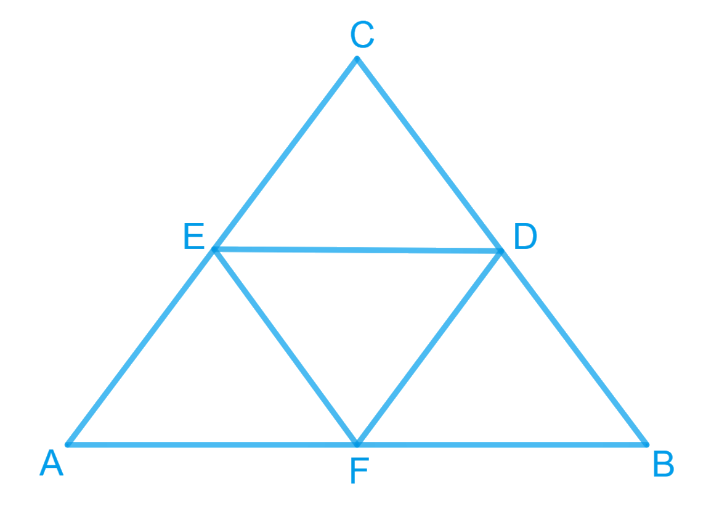# Ex.9.3 Q5 Areas of Parallelograms and Triangles Solution - NCERT Maths Class 9

Go back to  'Ex.9.3'

## Question

$$D$$, $$E$$ and $$F$$ are respectively the mid-points of the sides $$BC$$, $$CA$$ and $$AB$$ of a $$\Delta ABC$$. Show that:

(i) $$BDEF$$ is a parallelogram

(ii) \begin{align}{ar } (DEF) = \frac { 1 } { 4 } { ar } (ABC)\end{align}

(iii) \begin{align}{ar } (BDEF) = \frac { 1 } { 2 } { ar } (ABC)\end{align}

Video Solution
Areas Of Parallelograms And Triangles
Ex 9.3 | Question 5

## Text Solution

What is known?

$$D$$, $$E$$ and $$F$$ are respectively the mid-points of the sides $$BC$$, $$CA$$ and $$AB$$ of a $$\Delta \text{ABC}$$

What is unknown?

How we can show that

(i) $$BDEF$$ is a parallelogram.

(ii)$${ar }\left( {DEF} \right){ = }\frac{{1}}{{4}}{ar }\left( {ABC} \right)$$

(iii) $${ar }\left( {BDEF} \right){ = }\frac{{1}}{{2}}{ar }\left( {ABC} \right)$$

Reasoning:

Line joining the mid-points of two sides of a triangle is parallel to the third and half of its length. If one pair of opposite side in quadrilateral is parallel and equal to each other then it is a parallelogram. Diagonals divides the parallelogram in two triangles of equal areas.

Steps:

(i) $$F$$ is the mid-point of $$AB$$ and $$E$$ is the mid-point of $$AC$$.

\begin{align} \therefore \; FE \| BC \text { and } FE = \frac { 1 } { 2 } BC \end{align}Line joining the mid-points of two sides of a triangle is parallel to the third and half of its length.

\begin{align} \therefore FE \| BD \,[BD \text { is the part of } BC]\end{align}

\begin{align} \text { Also, } D \text { is the mid-point of BC. }\end{align}

$\therefore {{BD = }}\frac{{{1}}}{2}{{ BC}}$

\begin{align} FE = BD\end{align}

Now  $$FE\|BD$$ and $$FE = BD$$

Since one pair of opposite side in quadrilateral $$BDEF$$ is parallel and equal to each other

Therefore, $$BDEF$$ is a parallelogram.

(ii) $$BDEF$$ is a parallelogram.

\begin{align} \therefore { ar } (\Delta BDF) = {ar } (\Delta DEF) \ldots (i) \end{align}

[The diagonal $$DF$$ of the parallelogram $$BDEF$$ divides it in two triangles $$BDF$$ and $$DEF$$ of equal area]

Similarly,$$DCEF$$ is also parallelogram.

\begin{align} \therefore { ar } (\Delta DEF)\!=\! {ar } (\Delta DEC)\; \ldots (ii)\end{align}

Also, $$AEDF$$ is a parallelogram.

\begin{align} \therefore\!{ ar } (\Delta AFE)\!=\!{ar } (\Delta DEF) \;\ldots (iii)\end{align}

From equations (i), (ii) and (iii),

\begin{align} (\Delta DEF)& = { ar } (\Delta BDF)\\&= { ar } (\Delta DEC) \\&= { ar } (\Delta AFE)\;\ldots (iv) \end{align}

Now,

\begin{align}&{ar}(\Delta ABC)\\&\!=\!\left[ \begin{array} {ar}(\Delta DEF)\!+\!{ar}(\Delta BDF)+ \\ {ar}(\Delta DEC)\!+\!{ar}(\Delta {AFE})\ \\\end{array} \right]\!\ldots\! ({v})\end{align}

\begin{align}&{{ar}}(\Delta ABC)\\&\!=\!\left[ \begin{array}{l}{{ ar }}(\Delta DEF)\!+\!{{ ar }}(\Delta DEF)\!+\!\\{{ ar }}(\Delta DEF)\!+\!{{ ar }}(\Delta DEF)\end{array} \right]\end{align}

$${\left[ {{{Using }}\left( {{{iv}}} \right){{ \& }}\left( {{v}} \right)} \right]}$$

\begin{align} & {ar } (\Delta ABC) = 4 \times { {ar } (\Delta DEF) } \\ & {ar } (\Delta DEF) = \frac { 1 } { 4 } { ar } (\Delta ABC) \end{align}

(iii)

\begin{align} {ar } (\| gm\; BDEF) &= {ar } (\Delta BDF) + {ar } (\Delta DEF)\\&= {ar } ( \Delta DEF) + {ar } (\Delta DEF) \\\text{ Using } (iv) \end{align}

\begin{align} {ar } (\| { gm } BDEF) &\!=\!2 { ar }\!\!(\Delta DEF) \\ { ar } (\| { gm } BDEF) &\!=\! 2\!\times\!\frac { 1 } { 4 }\!\! { ar }\!\!(\Delta ABC) \\\! { ar }\!( \| { gm } BDEF) &\!=\! \frac { 1 } { 2 } { ar }\!(\Delta ABC) \end{align}

Video Solution
Areas Of Parallelograms And Triangles
Ex 9.3 | Question 5